Life is quite disrupted during this hard time of pandemic. It is especially stressful to see new cases rising again within the U.S.. When will the pandemic come to its peak? I belive many have asked about this question and many have given it serious thought. In this blog post, I want to give this question an answer using SIR model.

import numpy as np
import pandas as pd
import scipy
from scipy import integrate
from scipy import optimize

import matplotlib.pyplot as plt

import platform
import datetime

print(f'''I use Python {platform.python_version()} with:
numpy {np.__version__}
pandas {pd.__version__}
scipy {scipy.__version__}
''')

I use Python 3.7.3 with:
numpy 1.17.2
pandas 0.25.1
scipy 1.4.1



## SIR model

SIR model is a simple yet powerful model to describe an epidemic. It separates a population in a certain region into 3 parts: Susceptible ($S(t)$), Infected ($I(t)$) and Removed ($R(t)$, either die or recover from the epidemic). The SIR model is then modeled by the following system of differential equations:

\begin{align*} \frac{dS}{dt} &= -\frac{\beta SI}{N}, \\ \frac{dI}{dt} &= \frac{\beta SI}{N} - \gamma I, \\ \frac{dR}{dt} &= \gamma I, \end{align*}

where $N = S(t) + I(t) + R(t)$ is the constant of total population, $\beta$ is the contact rate, and $\gamma$ is the removed rate.

def sir_diff(t, y, beta, gamma):
S, I, R = y
N = sum(y)

dS = -beta * S * I / N
dI = -dS - gamma * I
dR = gamma * I

return dS, dI, dR

def sir(t_eval, y0, beta, gamma):
result = integrate.solve_ivp(
sir_diff,
[t_eval, t_eval[-1]],
y0,
t_eval=t_eval,
args=(beta, gamma)
)
return result.y


Let's simulate a scenario: suppose the total population $N = 10000$ with $\beta = 0.2$ and $\gamma = 0.1$. Initially, we have $S(0) = 9999, I(0) = 1$ and $R(0) = 0$. We simulate 300 points.

points = np.arange(300)
y0 = [9999, 1, 0]
beta = 0.2
gamma = 0.1
S, I, R = sir(points, y0, beta, gamma)

plt.plot(points, S, label="Susceptible")
plt.plot(points, I, label="Infected")
plt.plot(points, R, label="Removed")
plt.title("SIR with beta=0.2, gamma=0.1")
plt.legend()
plt.show()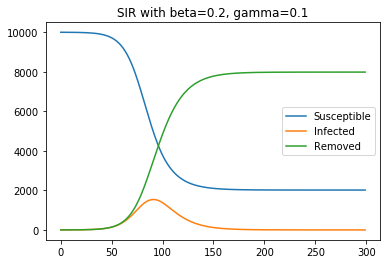Based on the figure above, we can say that the simulated scenario ends its epidemic around $t = 150$. We hope to find a good set of parameters $\beta$ and $\gamma$ for the COVID-19 in the United States, so that we can spot its end in a similar figure.

## COVID-19 data

I am going to use COVID-19 Data Repository by the Center for Systems Science and Engineering (CSSE) at Johns Hopkins University. In the country level, we can find confirmed cases, death cases (along with total population) and recovered cases in this repository. In the state level, we can only find confirmed cases and death cases (along with total population). Here,

confirmed cases = Infected + Removed

Removed = death cases + recovered cases.

So for the United States, we can get time series for $I(t)$ and $R(t)$, and thus $S(t)$. Since $S$ is redundant if we know $I$ and $R$, we use only $I$ and $R$ to train the model. For state level, we can not get $I$ and $R$ sepepartely, only $I + R$ as confirmed cases. So it is a little bit differentent to train an SIR model for a state.

### Confirmed cases

df = pd.read_csv("https://raw.githubusercontent.com/CSSEGISandData/COVID-19/master/csse_covid_19_data/csse_covid_19_time_series/time_series_covid19_confirmed_US.csv")
df = df[df.columns[11:]]
total = df.sum()

confirmed = total.to_numpy()
points = np.arange(len(confirmed))

plt.plot(points, confirmed)
plt.title("confirmed cases in the U.S.")
plt.show()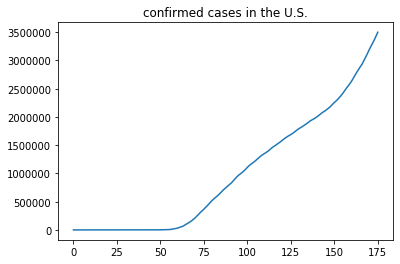### Deaths cases

df = pd.read_csv("https://raw.githubusercontent.com/CSSEGISandData/COVID-19/master/csse_covid_19_data/csse_covid_19_time_series/time_series_covid19_deaths_US.csv")
df = df[df.columns[12:]]
total = df.sum()

deaths = total.to_numpy()

plt.plot(points, deaths)
plt.title("deaths cases in the U.S.")
plt.show()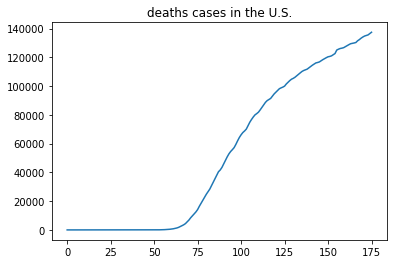### Recovered cases

df = pd.read_csv("https://raw.githubusercontent.com/CSSEGISandData/COVID-19/master/csse_covid_19_data/csse_covid_19_time_series/time_series_covid19_recovered_global.csv")
df = df[df["Country/Region"] == "US"][df.columns[4:]]
recovered = df.iloc.to_numpy()

plt.plot(points, recovered)
plt.title("recovered cases in the U.S.")
plt.show()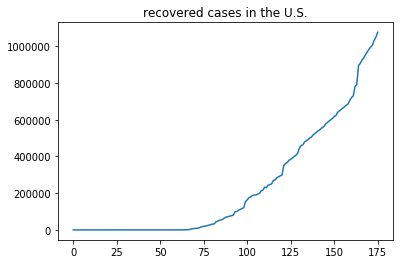### Population

Population data can be found in the deaths cases.

df = pd.read_csv("https://raw.githubusercontent.com/CSSEGISandData/COVID-19/master/csse_covid_19_data/csse_covid_19_time_series/time_series_covid19_deaths_US.csv")
population = df["Population"].sum()

print(f"Population of the U.S.: {population}.")

Population of the U.S.: 338752267.


### Time series for I, R

We don't need to compute the time series for $S(t)$, since it can be recovered from $I(t)$ and $R(t)$ easily.

R_observed = deaths + recovered
I_observed = confirmed - R_observed

plt.plot(points, I_observed, label="Observed Infected")
plt.plot(points, R_observed, label="Observed Removed")
plt.legend()
plt.show()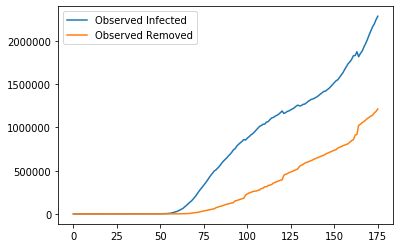## Maximum likelihood estimation

Let $C_t$ be the simulated cases (can be infected cases, removed cases or confirmed cases) by the SIR model, and $O_t$ be the observed cases. $C_t$ depends on the parameters $\beta$ and $\gamma$. We assume for simplicity that $O_t$ has the Poisson distribution with parameter $C_t$. Thus, the possibility of observing $O_t$ cases given simulated cases $C_t$ is

$$P(O_t; C_t) = \frac{C_t^{O_t}e^{-C_t}}{O_t!}.$$

Therefore, the likelihood function is

$$L(\beta, \gamma) = \prod_{t} \frac{C_t^{O_t}e^{-C_t}}{O_t!},$$

where $t$ runs over all time points.

Then the log likelihood function is

$$l(\beta, \gamma) = \sum_{t} O_t \log(C_t) - C_t - \log(O_t!).$$

We want paramters $\beta$ and $\gamma$ to maximize $l(\beta, \gamma)$. Since $O_t$ are constant, we just need to minimize the simplified log likelihood function

$$ll(\beta, \gamma) = -\sum_{t} O_t \log(C_t) - C_t.$$
def ll(params, N, I_obs, R_obs):
beta, gamma = params
S0 = N - I_obs - R_obs
y0 = S0, I_obs, R_obs
t_points = np.arange(len(I_obs))
S, I, R = sir(t_points, y0, beta, gamma)

# avoid 0 in I or R by adding a small epsilon
epsilon = np.exp(-10)
I = I + epsilon
R = R + epsilon

return -np.sum(I_obs * np.log(I) - I) - np.sum(R_obs * np.log(R) - R)


ll takes four parameters, params a 2-tuple $(\beta, \gamma)$ for the SIR model, N total population, I_obs the observed infected cases, and R_obs the observed removed cases.

## Result

We minimize ll using scipy.optimize.minimize.

res = optimize.minimize(
ll,
np.array([0.5, 0.5]),
args=(population, I_observed, R_observed),
bounds=[(0, 1), (0, 1)],
method="L-BFGS-B")
beta, gamma = res.x
print(f'''Parameters for SIR model:
beta = {beta:.4f}
gamma = {gamma:.4f}

Basic reproductive number: {beta / gamma:.2f}.
''')

Parameters for SIR model:
beta = 0.1400
gamma = 0.0486

Basic reproductive number: 2.88.


points = np.arange(len(I_observed))
S, I, R = sir(
points,
[population - confirmed, I_observed, R_observed],
beta, gamma
)
plt.plot(points, I, label="Simulated Infected")
plt.plot(points, I_observed, label="Observed Infected")
plt.plot(points, R, label="Simulated Removed")
plt.plot(points, R_observed, label="Observed Removed")
plt.legend()
plt.title("Simulated cases vs Observed cases")
plt.show()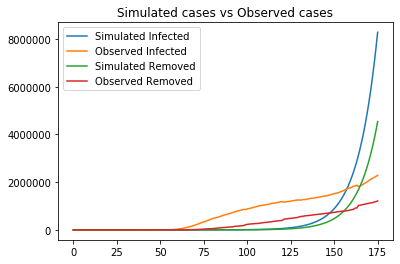From the figure above, the model is very far from perfect. There are a lot of possible reasons. Let me name some:

1. The SIR model fails to capture the long-term dynamic of COVID-19
2. Data collected are not accurate
3. Our algorithms are deficient to find the good parameters

To quantify the quality of the model, we are going to use the mean absolute percentage error (MAPE).

def mape(observed, predicted):
error = np.abs(observed - predicted)
observed = np.abs(observed) + np.exp(-4) # avoid 0
error = error / observed
return error.mean() * 100

I_err = mape(I_observed, I)
R_err = mape(R_observed, R)
print(f'''MAPE for Infected cases: {I_err:.2f}%
MAPE for Removed cases: {R_err:.2f}%
''')

MAPE for Infected cases: 83.04%
MAPE for Removed cases: 496.35%



The MAPE are high, another evidence for a bad SIR model. In order to get a better model, we limit ourselves to recent COVID-19 data instead of taking all data into consideration. I expect a better model because I belive that SIR at least capture the short-term dynamic of COVID-19 and that the data collected recently are more accurate than those collected at the early stage of the pandemic.

### 30 days' data

I will take recent 30 days' data to train the SIR model.

n = 30
I_recent = I_observed[-n:]
R_recent = R_observed[-n:]

res = optimize.minimize(
ll,
np.array([0.5, 0.5]),
args=(population, I_recent, R_recent),
bounds=[(0, 1), (0, 1)],
method="L-BFGS-B")
beta, gamma = res.x
print(f'''Parameters for SIR model:
beta = {beta:.4f}
gamma = {gamma:.4f}

Basic reproductive number: {beta / gamma:.2f}.
''')

Parameters for SIR model:
beta = 0.0247
gamma = 0.0093

Basic reproductive number: 2.65.


points = np.arange(n)
S, I, R = sir(
points,
[population - I_recent - R_recent, I_recent, R_recent],
beta, gamma
)
plt.plot(points, I, label="Simulated Infected")
plt.plot(points, I_recent, label="Observed Infected")
plt.plot(points, R, label="Simulated Removed")
plt.plot(points, R_recent, label="Observed Removed")
plt.legend()
plt.title("Simulated cases vs Observed cases")
plt.show()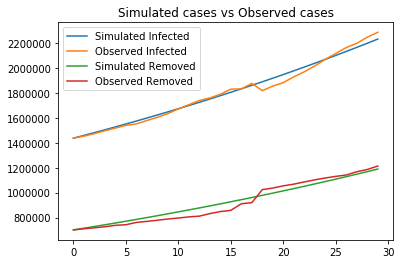I_err = mape(I_recent, I)
R_err = mape(R_recent, R)
print(f'''MAPE for Infected cases: {I_err:.2f}%
MAPE for Removed cases: {R_err:.2f}%
''')

MAPE for Infected cases: 1.22%
MAPE for Removed cases: 3.73%



Both figure and MAPE indicate a much better SIR model if we use only recent data. Now we can use this fitted model to project the future of the pandemic.

t_points = np.arange(1000)
S, I, R = sir(
t_points,
[population - I_recent - R_recent, I_recent, R_recent],
beta, gamma
)

plt.plot(t_points, I, label="Simulated Infected")
plt.plot(t_points, R, label="Simulated Removed")
plt.legend()
plt.title("Simulated cases")
plt.show()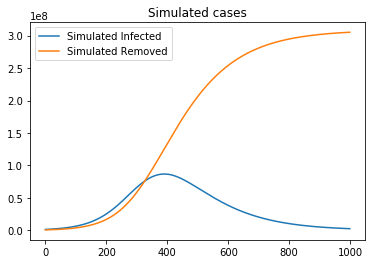Day 0 is the starting date of our simulation, which is 30 days ago. The figure above reads that COVID-19 reaches its peak at around day 400, which is roughly a year later from today!

today = datetime.date.today()
simulation_start_date = today - datetime.timedelta(days=30)
peak_day = int(np.argmax(I))
peak_date = simulation_start_date + datetime.timedelta(days=peak_day)

print(f"Peak at day {peak_day} on {peak_date}")

Peak at day 391 on 2021-07-12


We need to interpret the result correctly. According to the trend of most recent 30 days' data, the SIR model predicts that in the worse scenario where everyone gets the disease, COVID-19 reaches its peak on July 12, 2021. Don't panic. It is almost impossible to get into the worse scenario.

## Discussion

I want to see the peak dates of better scenarios. Hopefully, the result will cheer you and me up a bit. We can safely claim that not everyone will get COVID-19, due to our effort (wearing masks, keep social distance, and etc.). Let's see what the percentage of comfirmed cases among the whole population so far.

print(f"confirmed cases / population: {confirmed[-1] * 100 / population:.2f}%")

confirmed cases / population: 1.03%

def scenario(N, n_day=30, print_info=True):
I_recent = I_observed[-n_day:]
R_recent = R_observed[-n_day:]
points = np.arange(n_day)
res = optimize.minimize(
ll,
np.array([0.5, 0.5]),
args=(N, I_recent, R_recent),
bounds=[(0, 1), (0, 1)],
method="L-BFGS-B")
beta, gamma = res.x

y0 = [N - I_recent - R_recent, I_recent, R_recent]
S, I, R = sir(points, y0, beta, gamma)
I_err = mape(I_recent, I)
R_err = mape(R_recent, R)

future = np.arange(1000)
S, I, R = sir(future, y0, beta, gamma)
peak_day = int(np.argmax(I))
today = datetime.date.today()
simulation_start_date = today - datetime.timedelta(days=n_day)
peak_date = simulation_start_date + datetime.timedelta(days=peak_day)

if print_info:
print(f'''Parameters for SIR model (using most recent {n_day} days' data):
beta = {beta:.4f}
gamma = {gamma:.4f}

Basic reproductive number: {beta / gamma:.2f}

MAPE for Infected cases: {I_err:.2f}%
MAPE for Removed cases: {R_err:.2f}%

Peak at day {peak_day} on {peak_date}''')

return beta, gamma, peak_day, peak_date


Let's look at a scenario where only 5% of the total population get the COVID-19.

_ = scenario(population * 0.05)

Parameters for SIR model (using most recent 30 days' data):
beta = 0.0289
gamma = 0.0093

Basic reproductive number: 3.11

MAPE for Infected cases: 1.36%
MAPE for Removed cases: 3.74%

Peak at day 158 on 2020-11-21


The peak date is much eariler than the worse scenario. I list some scenarios below.

percentages = np.arange(0.02, 0.11, 0.01)
print("Scenario: Peak Date")
for p in percentages:
_, _, _, peak = scenario(population * p, print_info=False)
print(f"{p * 100:.1f}%: {peak}")

Scenario: Peak Date
2.0%: 2020-09-05
3.0%: 2020-10-08
4.0%: 2020-11-01
5.0%: 2020-11-21
6.0%: 2020-12-07
7.0%: 2020-12-21
8.0%: 2020-12-31
9.0%: 2021-01-10
10.0%: 2021-01-18


Each scenario represent the percentage of the total population that get the COVID-19. The smaller the percentage is, the better scenario we are in. Follow guidances from health authorities: wear masks, keep social distance, wash hands often and etc. That will help us get into a better scenario.